### Box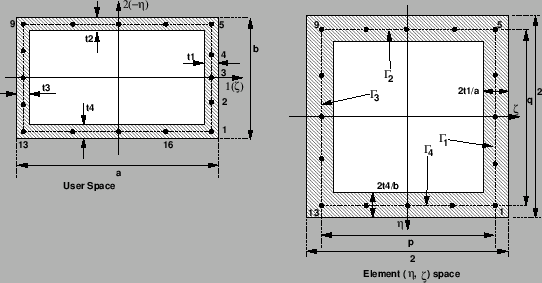The Box section (contributed by O. Bernhardi) is simulated using a 'parent' beam element of type B32R.

The outer cross sections are defined by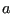and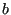, the wall thicknesses are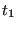,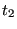,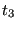and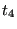and are to be given by the user (Figure 81).

The cross-section integration is done using Simpson's method with 5 integration points for each of the four wall segments. Line integration is performed; therefore, the stress gradient through an individual wall is neglected. Each wall segment can be assigned its own wall thickness.

The integration in the beam's longitudinal direction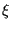is done using the usual Gauss integration method with two stations; therefore, the element has a total of 32 integration points.

From the figure, we define, for example, the local coordinates of the first integration point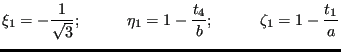(19)

The other three corner points are defined correspondingly. The remaining points are evenly distributed along the center lines of the wall segments. The length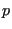and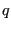of the line segments, as given w.r.t. the element intrinsic coordinates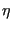and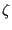, can now be calculated as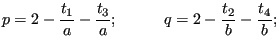(20)

An integral of a function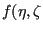), over the area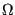of the hollow cross section and evaluated w.r.t the natural coordinates,, can be approximated by four line integrals, as long as the line segments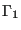,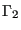,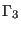and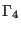are narrow enough: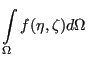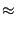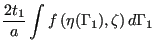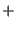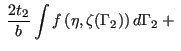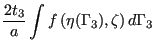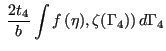(21)

According to Simpson's rule, the integration points are spaced evenly along each segment. For the integration weights we get, for example, in case of the first wall segment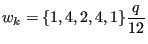(22)

Therefore, we get, for example, for corner Point 1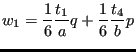(23)

and for Point 2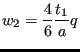(24)

The resulting element data (stresses and strains) are extrapolated from the eight corner integration points (points 1,5,9 and 13) from the two Gauss integration stations using the shape functions of the linear 8-node hexahedral element.

Remarks

• The wall thickness are assumed to be small compared to the outer cross section dimensions.
• The bending stiffnesses of the individual wall segments about their own neutral axes are completely neglected due to the line integral approach.
• Torsion stiffness is governed to a large extent by warping of the cross section which in turn can only be modelled to a limited extent by this type of element.
• Modelling of U or C profiles is also possible by setting one of the wall thicknesses to zero. Modelling L sections however, by setting the wall thickness of two segments to zero, will probably cause spurious modes.